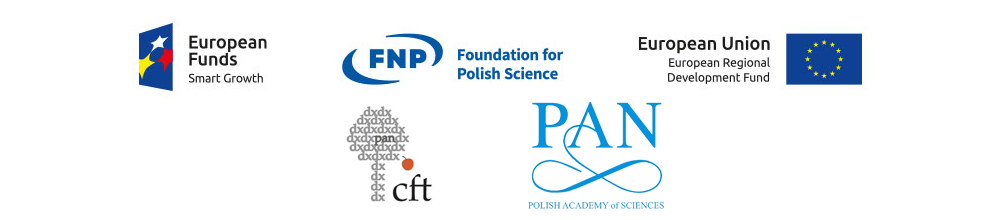Michał Oszmaniec Quantum Information Research Group
Quantin.pl is a website of the Quantum Information Group led by dr. Michał Oszmaniec. We study quantum information under the restrictions on resources that are allowed to be used. Such limitations arise from the difficulty to implement in practice the most general quantum-information protocols. In our research we use the combination of convex analysis, group theory, high-dimensional analysis and numerical simulations.
Michał Oszmaniec, michał, oszmaniec, quantum information, quantum, qbits, quantin
20145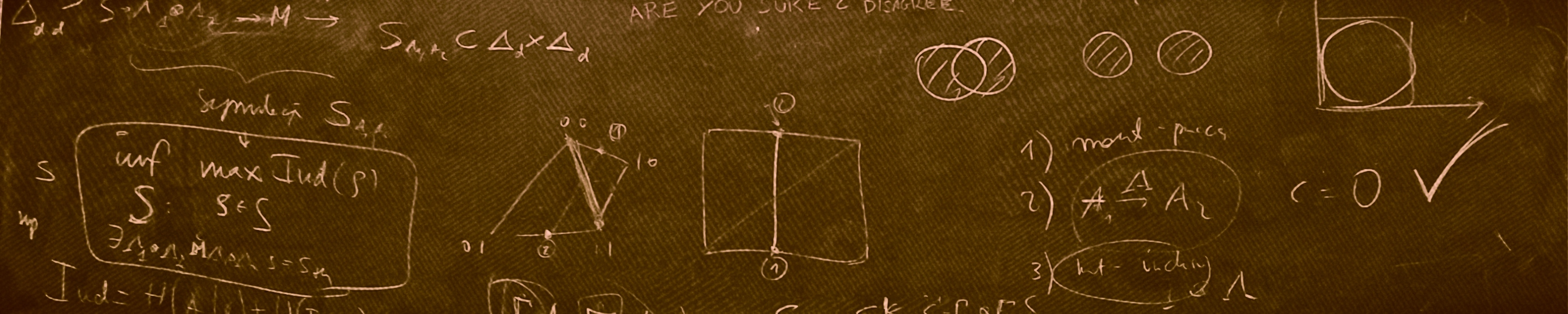THE TEAM

#### Meet the team: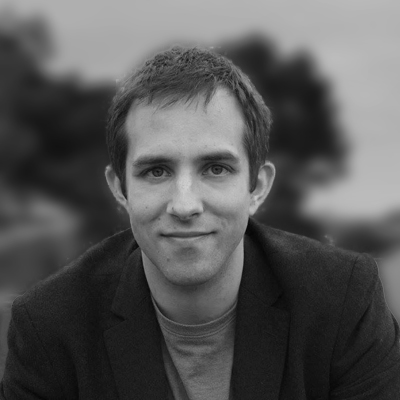##### MICHAŁ OSZMANIEC
Principal investigator

oszmaniec@cft.edu.pl

I am a theoretical physicist specialising in quantum technologies. My current scientific interests oscillate around quantum computing and quantum information, understood both on the fundamental, as well as on the practical level.
The main topics of my current research are:
• Role of various kinds of correlations (such as contextuality, non-gaussianity) for quantum computation,
• Characterisation and error mitigation in near-term quantum computers,
• Efficient classical simulation of noisy large-scale quantum systems, with applications to quantum supremacy and quantum simulations,
• Application of group-theoretic methods in conjunction of machine-learning techniques to derive new control schemes for moderate-sized quantum systems,
• Understanding the relative power and usefulness of POVMs and protective (von-Neumann) measurements in quantum mechanics.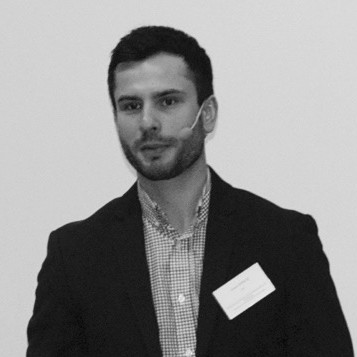Senior scientist

a.sawicki@cft.edu.pl

I am a mathematical/theoretical physicist specialising (among others) in quantum information theory. For a few last years I have been particularly interested in theoretical aspects of quantum computing.
The main topics of my current research are:
• Efficient quantum gates
• Variants of Solovay-Kitaev theorem
• Random quantum compilers
• t-designs and epsilon nets
• applications of control theory in quantum computing
• geometry of quantum correlations

.

.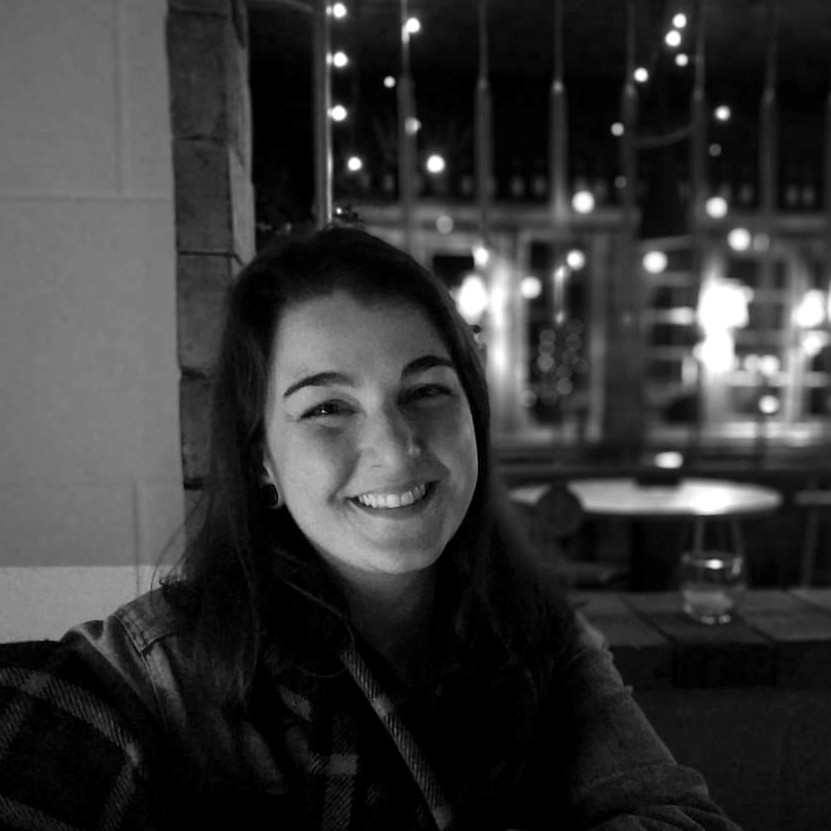##### Susane Calegari
PhD student

calegari@cft.edu.pl

My key areas of interest are classical simulation of quantum computers, quantum computational resources, quantum foundations, and quantum contextuality. I am a theoretical physicist with a master thesis on conformal invariance applied to quantum field theory and gravity. My research in quantum information has been on the understanding of the structure of correlations in multipartite systems. Now, as a Ph.D. student, I want to focus my studies on the role of contextuality as a resource for quantum computation.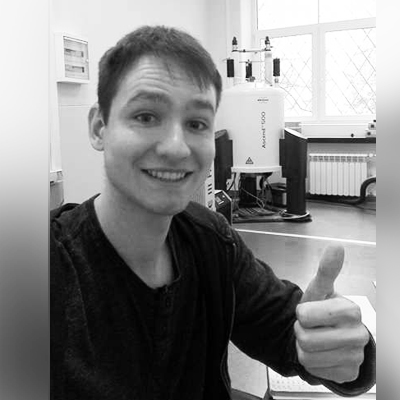##### Filip Maciejewski
PhD student

maciejewski@cft.edu.pl

My scientific interests include both foundations of quantum information theory and possible applications on near-term quantum devices. I am particularly interested in the theory of generalized quantum measurements (POVMs).

My research as a PhD student includes:

• characterization of measurement noise and development of methods for its mitigation;
• study of relative power of POVMs and projective measurements in various quantum information protocols;
• development of new schemes of implementation of POVMs.

.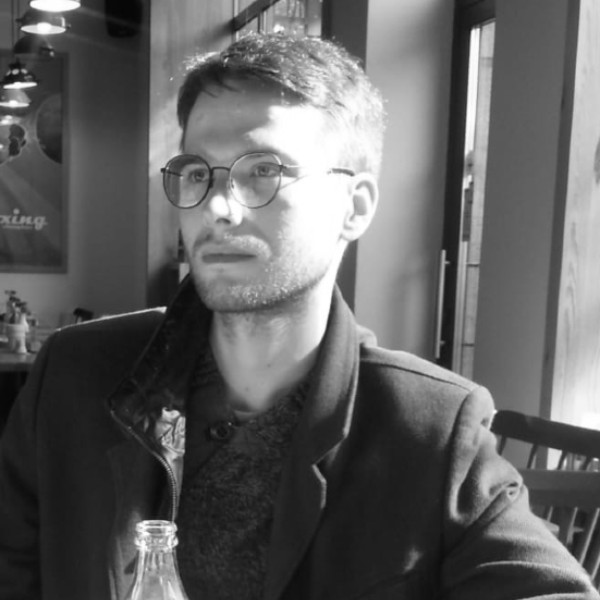##### Oskar Słowki
Project student

oslowik@cft.edu.pl

My scientific interests span a wide range of topics from theoretical computer science, through theoretical physics to pure mathematics. Quantum information and computation lies at the intersection of those three. My main area of research is mathematical physics, specifically applications of geometry and topology in quantum information and computation.

My research within the group focuses on:
– entanglement in multipartite systems and its geometry
– efficient quantum gates and compilers
– projective simulations of generalized quantum measurements
– quantum walks on graphs##### Jakub Reszke
Project student

jm.reszke@student.uw.edu.pl

I am interested in exploring variety of topics within quantum information theory. My work focuses on applying group-theoretic methods to problem of efficient classical simulatability of quantum computation.